# Group completion

Given a topological monoid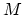, the group completion is the space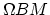, where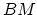is the classifying space of(thinking ofas a topological category). Ifis already a topological group, then this operation does not changeup to homotopy equivalence. Under some assumptions, we have the following description of the homology of the group completion. If we treat the monoid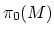as a directed system (with maps given by the monoid operation), then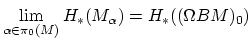in situations where the direct limit on the left-hand side is well-defined. In particular, if we consider the monoid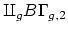, the homology of the group completion is precisely the stable homology. The plus construction can often be used to give an alternative construction of the group completion.

Jeffrey Herschel Giansiracusa 2005-06-27